If a current flowing through a lightbulb is 0.75 ampere and the voltage difference across the lightbulb is 120 volts, how much resistance do

Question

If a current flowing through a lightbulb is 0.75 ampere and the voltage difference across the lightbulb is 120 volts, how much resistance does the light bulb have

in progress 0
6 months 2021-07-15T10:29:33+00:00 1 Answers 30 views 0

1. Answer: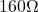Explanation:

According to Ohm’s law: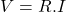Where: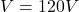is the voltage difference across the light bulb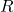is the resistance of the light bulb   (the value we want to find)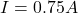is the electric current

Isolating: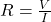Finally: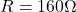This is the resistance of the light bulb